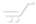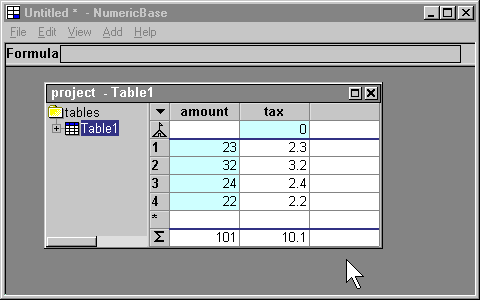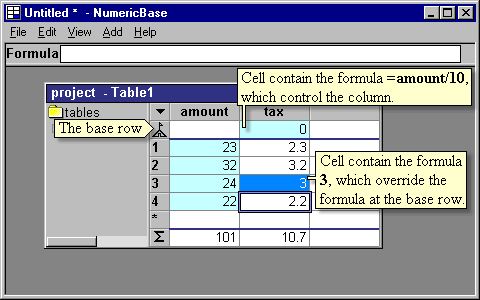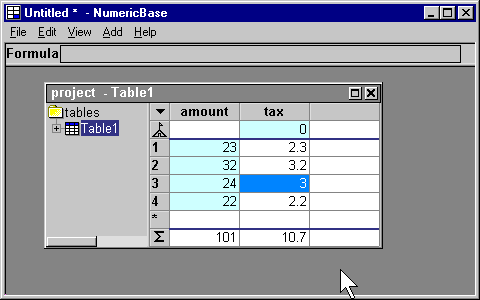# Overriding the base row

Overriding the base row
A formula at the base row controls all the values of the column. You can override this behavior for a specific cell. To do this, you can type your overriding formula at the desired cell - see the following animated screenshot for an example.Animated Screenshot: Overriding by entering a formula at a cell.

After we entered the formula at the cell, NumericBase uses this formula, instead of the formula at the base row, to calculates the value for the cell, as shown in the following screenshot.Screenshot: NumericBase uses the overriding formula.

Remove the overriding formula.
To remove this overriding formula, simply delete the formula from the cell - as shown in the following animated screenshot.Animated screenshot: removing the overriding formula from the cell